Computer Science

# Fix the bridgeThe black squares represent the ground. The blue squares represent water in a river. The red squares represents a bridge — except it is not complete!

Your job is to fix the bridge so that it looks like this:## Starter code

Download the zip file above and put it in your `bit` folder. You will find a file called `fix_bridge.py` that has the following starter code:

``````from byubit import Bit

@Bit.worlds('fix-bridge', 'fix-another-bridge')
def go_fix_the_bridge(bit):
# Write code here
pass

if __name__ == '__main__':
go_fix_the_bridge(Bit.new_bit)
``````

Notice that there is another world (`fix-another-bridge`) so your solution will need to be general enough to solve both problems:## Planning

Before you write any code, plan out your solution with pencil and paper. Find a friend and draw out how you think you would solve this problem.What did you draw? Maybe you drew something like this: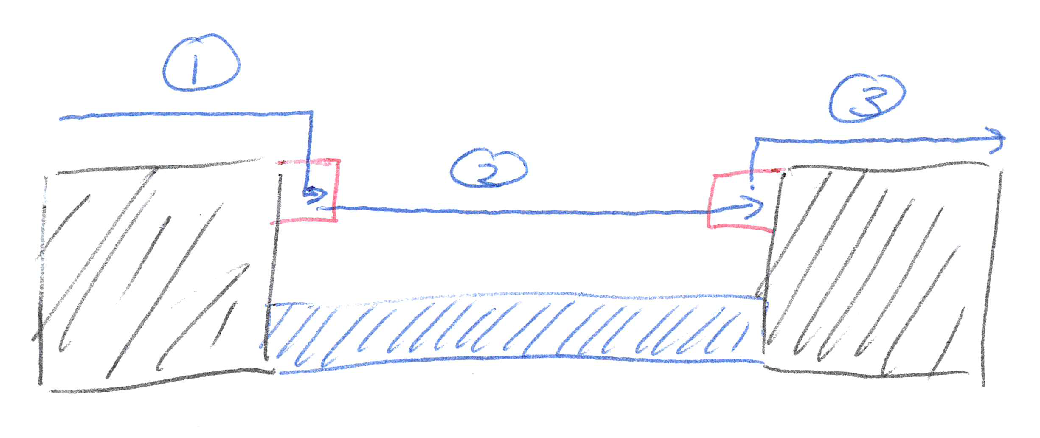• Step 1: Move to the bridge
• Step 2: Fix the bridge
• Step 3: Move off the bridge

## Decomposing the problem

Now start at the highest or most abstract level and write functions. For example:

``````def go_fix_the_bridge(bit):
""" Move to the bridge, fix the bridge, and move off the bridge. """
move_to_the_bridge(bit)
fix_the_bridge(bit)
move_off_the_bridge(bit)``````

Remember, PyCharm will put squiggly red lines underneath the functions you haven’t defined yet: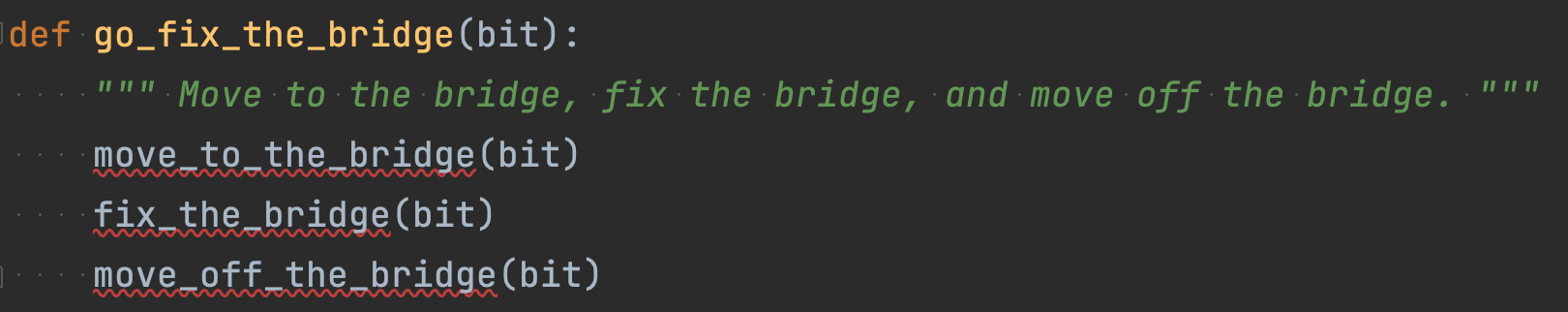Hover over these and select `Create function` in blue to create empty functions for each of them:

``````
def move_to_the_bridge(bit):
pass

def fix_the_bridge(bit):
pass

def move_off_the_bridge(bit):
pass

@Bit.worlds('fix-another-bridge', 'fix-bridge')
def go_fix_the_bridge(bit):
""" Move to the bridge, fix the bridge, and move off the bridge. """
move_to_the_bridge(bit)
fix_the_bridge(bit)
move_off_the_bridge(bit)``````

We define these functions with `pass` so that we can fill them in one at a time.

## Moving to the bridge

How would you move to the bridge?Here is one way to do this:

``````def move_to_the_bridge(bit):
""" Move to the left corner of the bridge.
End up on the red square, facing right
"""
while not bit.right_clear():
bit.move()

# Bit is now over the left end of the bridge
# Move down and onto the bridge, end facing to the right
bit.right()
bit.move()
bit.left()``````

We can use `while not bit.right_clear()` to keep moving until we get past the edge of the ground. This leaves us right above the red square. The last step is to turn, move down, and then turn back so we end up facing right. This puts Bit in good position to fix the bridge.

Stop and run your code to be sure it works: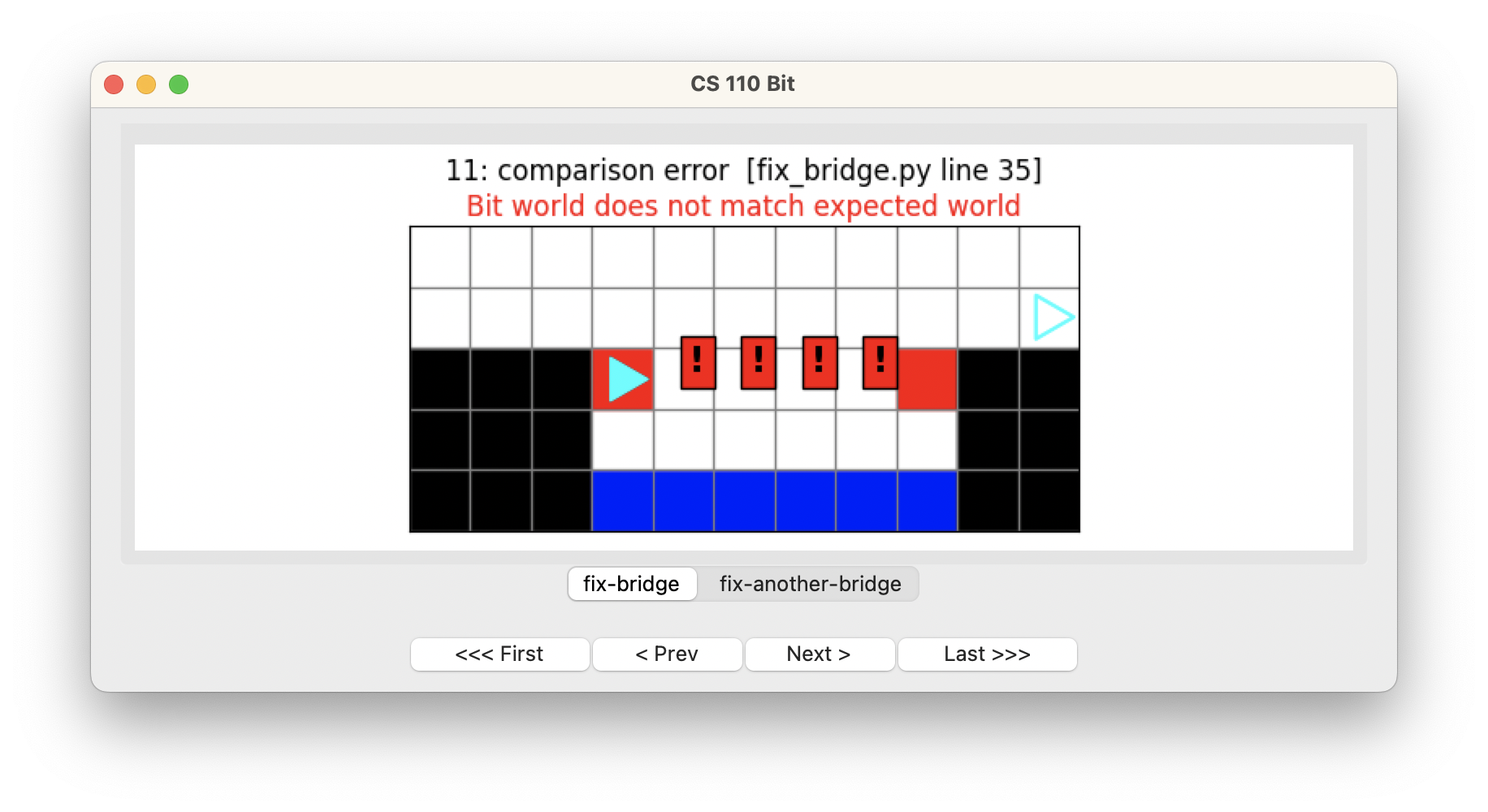It is OK that we haven’t solved the entire problem! We have the first step working great!

In the code above, you will see that there are some comments, which are lines starting with a pound sign `#`. Comments are a way to write notes to yourself and to others to explain what you were thinking when you wrote your code. They are really helpful!

Try to write comments liberally in your code. This will seem like extra work at first, but they will save you time as you write code. You will invariably take a break and come back to your code at a later time. Comments will let you know what you were thinking, especially if you are looking for bugs.

## Fix the bridge

OK, back to coding. How would you fix the bridge?Here is one way to do this:

``````def fix_the_bridge(bit):
""" Fix the bridge. End up on the last square, facing right. """
# move off the first red square
bit.move()
# now move until we get to a red square, painting as we go
while not bit.is_red():
bit.paint('red')
bit.move()``````

We want to use `while not bit.is_red()` to move to the right side of the bridge. But Bit starts on a red square! So we need to move Bit one square first, and then run this while loop.

Inside the while loop, we paint first and then move. If we move first and then paint, we would skip a square.

Let’s run our code, which covers the first two steps: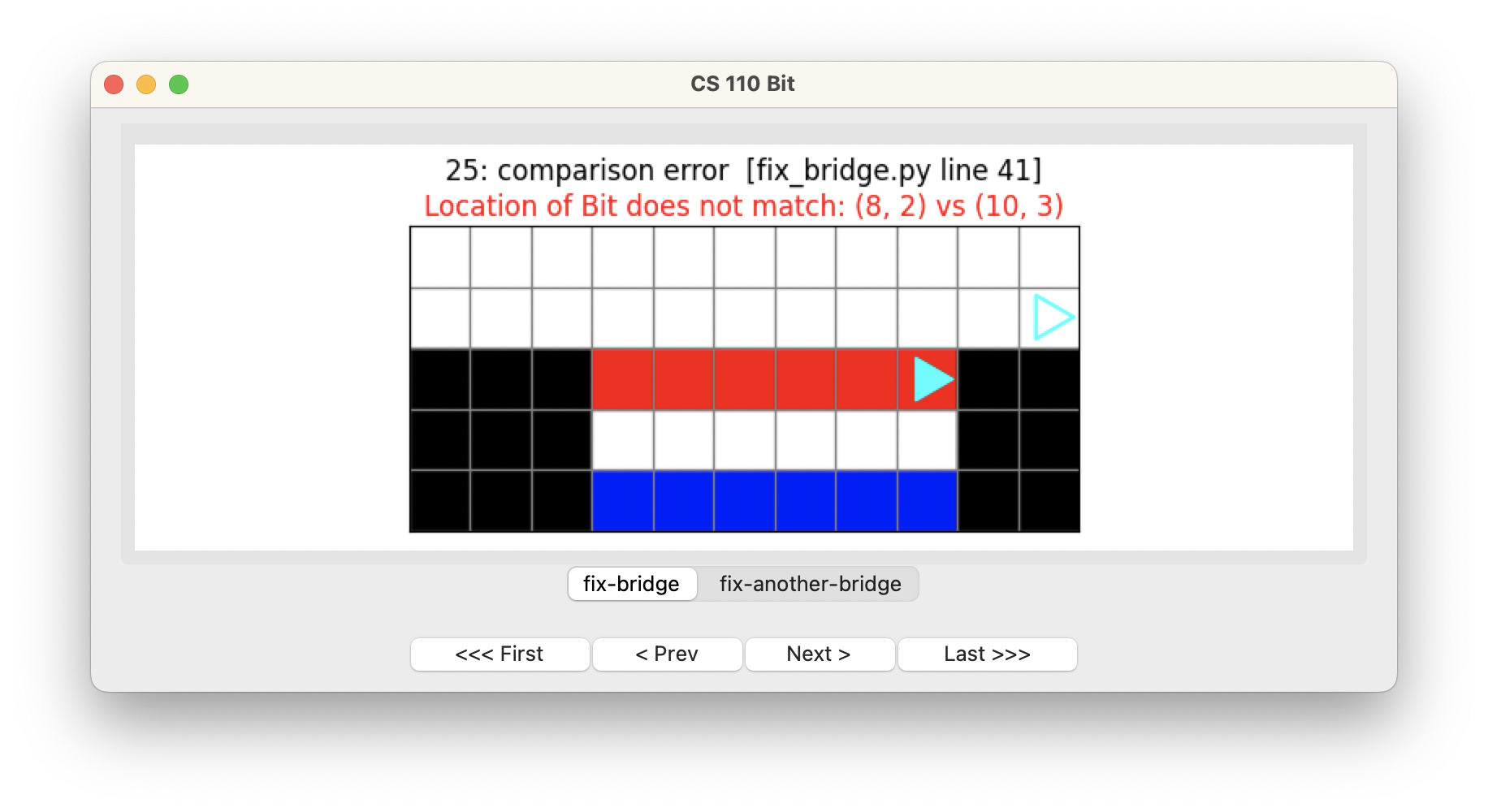We have made great progress! We have only one more step left.

## Move off the bridge

How would you move off the bridge and get Bit to its final position?Here is one way to do this:

``````def move_off_the_bridge(bit):
""" Move off the bridge and into the final position, at the right
edge of the world, facing right.
"""
# move off the bridge
bit.left()
bit.move()
bit.right()

# now move until Bit gets to the edge of the world
while bit.front_clear():
bit.move()``````

We first have to move Bit off the bridge. Once Bit is on the ground, we can use a while loop to move to the right edge of the world.

Let’s check to be sure this works: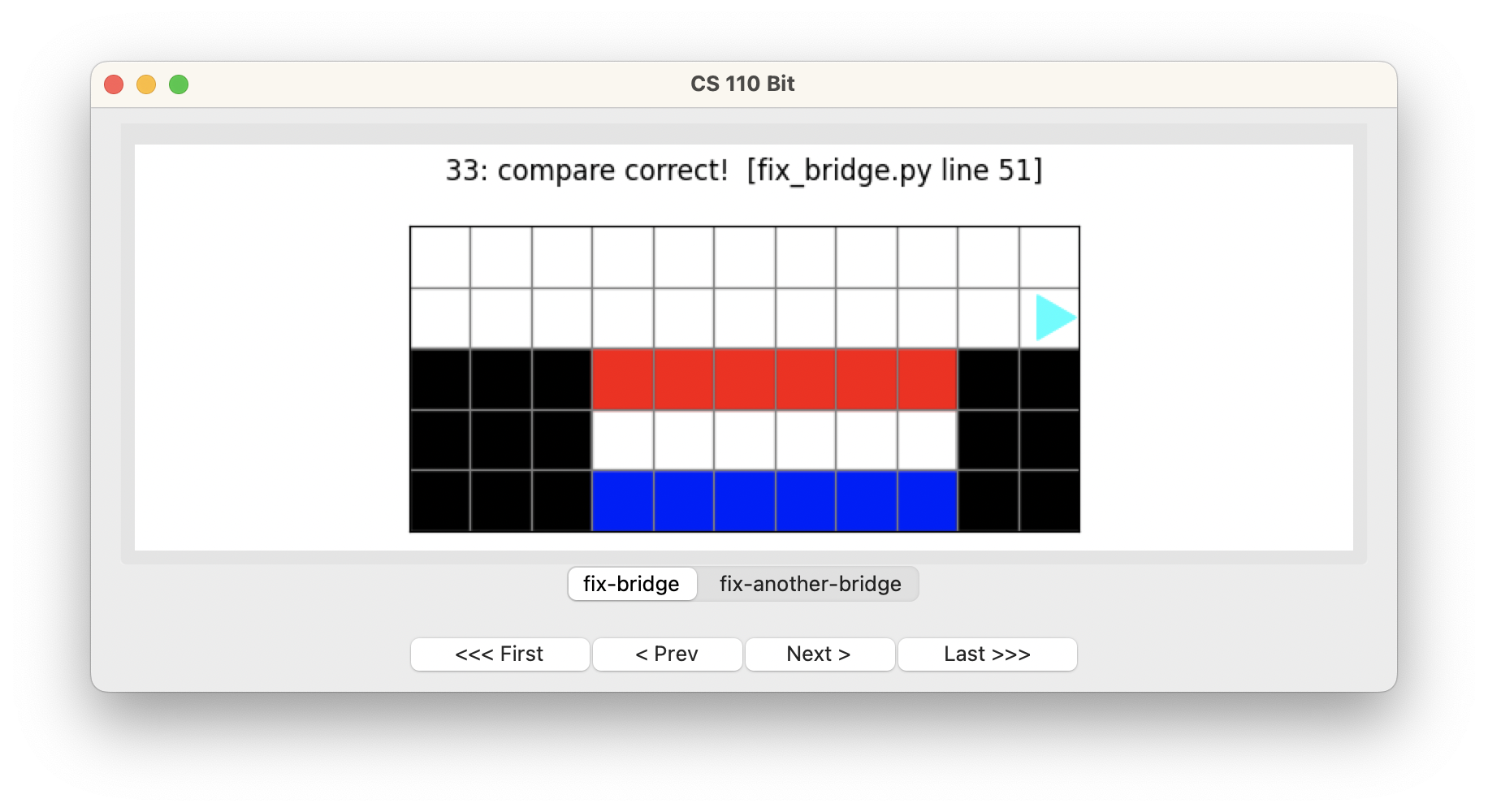Wonderful! Another problem solved!

## While versus while not

Notice how we sometimes use `while` and sometimes `while not`. To move to the edge of the bridge we use `while not`:

``````while not bit.right_clear():
bit.move()``````

We are looking for a clear space, so we need to move while it is not clear on the right.

However, to move to the edge of the world, we use just `while`:

``````while bit.front_clear():
bit.move()``````

This is because we are looking for a space that is not clear, so we need to move while it is clear.

With practice, this will become natural.